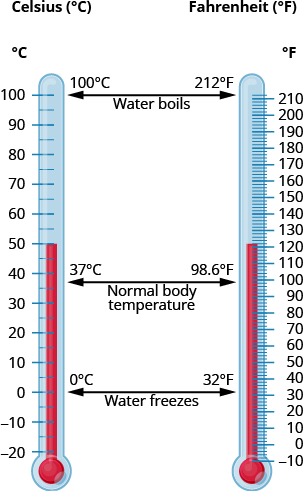## Converting Between U.S. and Metric Systems of Measurement

### Learning Outcomes

• Convert between the U.S. and the metric systems of measurement
• Convert between Fahrenheit and Celsius temperatures

Many measurements in the United States are made in metric units. A drink may come in $\text{2-liter}$ bottles, calcium may come in $\text{500-mg}$ capsules, and we may run a $\text{5-K}$ race. To work easily in both systems, we need to be able to convert between the two systems.

The reference table below shows some of the most common conversions.

Conversion Factors Between U.S. and Metric Systems
Length Weight Volume
$1$ in = $2.54$ cm

$1$ ft = $0.305$ m

$1$ yd = $0.914$ m

$1$ mi = $1.61$ km

$1$ m = $3.28$ ft

$1$ lb = $0.45$ kg

$1$ oz = $28$ g

$1$ kg = $2.2$ lb

$1$ qt = $0.95$ L

$1$ fl oz = $30$ mL

$1$ L = $1.06$ qt

We make conversions between the systems just as we do within the systems—by multiplying by unit conversion factors.

### example

Lee’s water bottle holds $500$ mL of water. How many fluid ounces are in the bottle? Round to the nearest tenth of an ounce.

Solution

 $500\text{mL}$ Multiply by a unit conversion factor relating mL and ounces. $500\text{mL}\cdot\Large\frac{1\text{fl oz}}{30\text{mL}}$ Simplify. $\Large\frac{500\text{fl oz}}{30}$ Divide. $16.7\text{fl. oz.}$ The water bottle holds $16.7$ fluid ounces.

### try it

The conversion factors in the reference table are not exact, but the approximations they give are close enough for everyday purposes. In the last example, we rounded the number of fluid ounces to the nearest tenth.

### Exercises

Soleil lives in Minnesota but often travels in Canada for work. While driving on a Canadian highway, she passes a sign that says the next rest stop is in $100$ kilometers. How many miles until the next rest stop? Round your answer to the nearest mile.

### Convert Between Fahrenheit and Celsius Temperatures

Have you ever been in a foreign country and heard the weather forecast? If the forecast is for $22^\circ C$. What does that mean?

The U.S. and metric systems use different scales to measure temperature. The U.S. system uses degrees Fahrenheit, written $^\circ F$. The metric system uses degrees Celsius, written $^\circ C$. The image below shows the relationship between the two systems.

A temperature of $37^\circ C$ is equivalent to $98.6^\circ F$.If we know the temperature in one system, we can use a formula to convert it to the other system.

### Temperature Conversion

To convert from Fahrenheit temperature, $\text{F}$, to Celsius temperature, $\text{C}$, use the formula

$C=\Large\frac{5}{9}\normalsize\left(F - 32\right)$
To convert from Celsius temperature, $\text{C}$, to Fahrenheit temperature, $\text{F}$, use the formula

$F=\Large\frac{9}{5}\normalsize C+32$

### Exercises

Convert $50^\circ F$ into degrees Celsius.

### example

The weather forecast for Paris predicts a high of $20^\circ C$ Convert the temperature into degrees Fahrenheit.

## Contribute!

Did you have an idea for improving this content? We’d love your input.